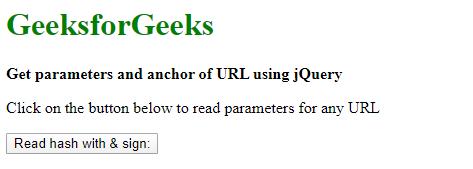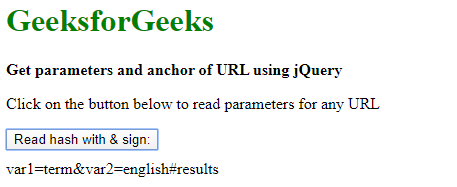It’s a very common situation in web development where users want to extract or read information from any URL. In the case of the unavailability of the server-side code, the programmer can make use of JavaScript or jQuery to read information. To read the URL with an & sign, we can use the split() function. By that function, we can easily get the URL parameter as a string. The programmer can retrieve the parameter string and store it in a variable by using JavaScript.

Syntax:

`var q = url.split('?');`

Here URL is any example URL string. To get URL query string parameters. If the URL has many parameters in the query string, the following code snippet will guide you to parse and store in variables that can be accessed.

```var vars = [], hash;
var q = url.split('?');
var fullParameter = q;

if(q != undefined) {
q = q.split('&');

for(var i = 0; i < q.length; i++) {
hash = q[i].split('=');
vars.push(hash);
vars[hash] = hash;
}

// Get hash parameter
var hashParameter = hash;
var hashstr= hashParameter.split('#');
}
```

To use any parameters, the user can access the value by parameter name. For example, if the URL contains the query string “?var1=name&var=surname&var3=address?, the user can access the value for “var1” using:

`alert(vars['var1']);`

Below example illustrates the above approach:

Example: This example reads URL parameters which includes hash along with "&" sign in the given URL.

 ` ` `<``html``> ` ` `  `<``head``> ` `    ``<``title``> ` `        ``How to read a hash with ` `        ``an & sign in the URL? ` `    `` ` `     `  `    ``<``script` `src``= ` `"https://code.jquery.com/jquery-3.3.1.min.js"``> ` `    `` ` ` ` ` `  `<``body``> ` `    ``<``h1` `style``=``"color: green"``> ` `        ``GeeksforGeeks ` `    `` ` `     `  `    ``<``b``> ` `        ``Get parameters and anchor ` `        ``of URL using jQuery ` `    `` ` `     `  `    ``<``p``> ` `        ``Click on the button below to ` `        ``read parameters for any URL ` `    `` ` ` `  `    ``<``button` `id``=``"btn"``> ` `        ``Read hash with & sign: ` `    `` ` `     `  `    ``<``div` `style``=``"height:10px;"``> ` ` `  `    ``<``div` `id``=``"Ampdiv"``> ` `     `  `    ``<``script``> ` `        ``var url =  ` `'http://www.geeksforgeeks.com/search?var1=term&var2=english#results'; ` `        ``\$('#btn').click(function() { ` `            ``readHash(url); ` `        ``}); ` ` `  `        ``function readHash(url) { ` `            ``var vars = [], hash; ` `            ``var q = url.split('?'); ` `            ``var fullParameter = q; ` ` `  `            ``if (q != undefined) { ` `                ``q = q.split('&'); ` ` `  `                ``for (var i = 0; i < ``q.length``; i++) { ` `                    ``hash` `= ``q``[i].split('='); ` `                    ``vars.push(hash); ` `                    ``vars[hash] = hash; ` `                ``} ` `                 `  `                ``// Get hash parameter ` `                ``var ``hashParameter` `= hash; ` `                ``var ``hashstr` `= ``hashParameter``.split('#'); ` `                ``alert("# Parameter :" + hashstr); ` `            ``} ` `             `  `            ``\$("#Ampdiv").text(fullParameter); ` `        ``} ` `    `` ` ` ` ` `  ` `

Output:

• Before clicking the button:• After clicking the button:My Personal Notes arrow_drop_upCheck out this Author's contributed articles.

If you like GeeksforGeeks and would like to contribute, you can also write an article using contribute.geeksforgeeks.org or mail your article to contribute@geeksforgeeks.org. See your article appearing on the GeeksforGeeks main page and help other Geeks.

Please Improve this article if you find anything incorrect by clicking on the "Improve Article" button below.